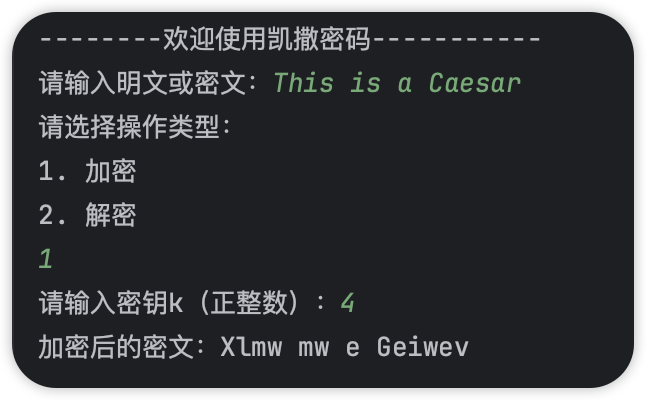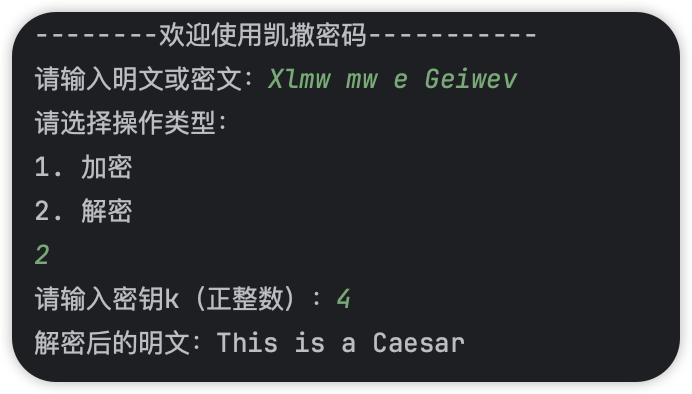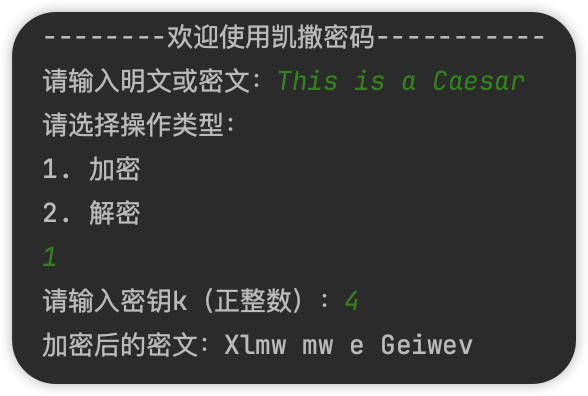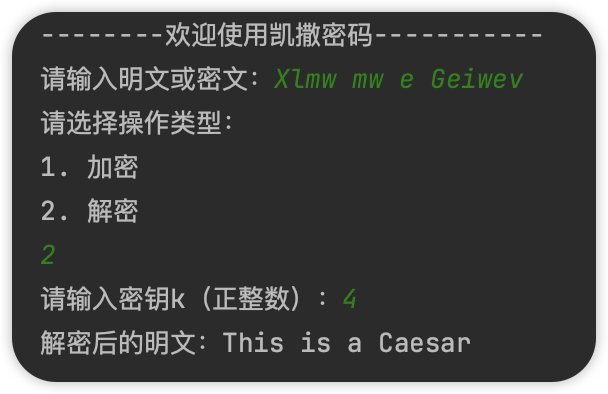# 3、公式

• 加密公式： f(a)=(a+N) mod 26
• 解密公式： f(a)=(a+(26-N)) mod 26

# 4、代码实现

## 4.1、C语言版本

C语言实现的凯撒密码的加解密

#include <stdio.h>
#include <string.h>
#include <ctype.h>

// 加密
void caesarEncrypt(char *text, char *result, int k) {
int length = strlen(text);
for (int i = 0; i < length; i++) {
char ch = text[i];
if (isalpha(ch)) {
char base;
if (islower(ch)) {
base = 'a';
} else {
base = 'A';
}
result[i] = (ch - base + k) % 26 + base;
} else {
result[i] = ch;
}
}
}

// 解密
void caesarDecrypt(char *text, char *result, int k) {
int length = strlen(text);
for (int i = 0; i < length; i++) {
char ch = text[i];
if (isalpha(ch)) {
char base;
if (islower(ch)) {
base = 'a';
} else {
base = 'A';
}
result[i] = (ch - base - k + 26) % 26 + base;
} else {
result[i] = ch;
}
}
}

int main() {
char text;
char result;
int k;
int type;

printf("--------欢迎使用凯撒密码-----------\n");
printf("请输入明文或密文：");
scanf("%[^\n]", text);

printf("请选择操作类型：\n");
printf("1. 加密\n");
printf("2. 解密\n");
scanf("%d", &type);

printf("请输入密钥k（正整数）：");
scanf("%d", &k);

if (type == 1) {
caesarEncrypt(text, result, k);
printf("加密后的密文：%s\n", result);
} else if (type == 2) {
caesarDecrypt(text, result, k);
printf("解密后的明文：%s\n", result);
} else {
printf("无效的操作类型。\n");
}

return 0;
}


1、加密验证2、解密验证## 4.2、C++版本

#include <iostream>
#include <string>
#include <cctype>

void caesarEncrypt(std::string& text, std::string& result, int k) {
for (char ch : text) {
if (std::isalpha(ch)) {
char base = std::islower(ch) ? 'a' : 'A';
result += static_cast<char>((ch - base + k) % 26 + base);
} else {
result += ch;
}
}
}

void caesarDecrypt(std::string& text, std::string& result, int k) {
for (char ch : text) {
if (std::isalpha(ch)) {
char base = std::islower(ch) ? 'a' : 'A';
result += static_cast<char>((ch - base - k + 26) % 26 + base);
} else {
result += ch;
}
}
}

int main() {
std::string text;
std::string result;
std::string kString;
int k;
int type;

std::cout << "--------欢迎使用凯撒密码-----------" << std::endl;
std::cout << "请输入明文或密文：";
std::getline(std::cin, text);

std::cout << "请选择操作类型：" << std::endl;
std::cout << "1. 加密" << std::endl;
std::cout << "2. 解密" << std::endl;
std::cin >> type;

std::cout << "请输入密钥k（正整数）：";
std::cin >> kString;

try {
k = std::stoi(kString);
} catch (const std::exception& e) {
std::cout << "无效的密钥。" << std::endl;
return 1;
}

std::cin.ignore(); // 忽略之前的换行符

if (type == 1) {
caesarEncrypt(text, result, k);
std::cout << "加密后的密文：" << result << std::endl;
} else if (type == 2) {
caesarDecrypt(text, result, k);
std::cout << "解密后的明文：" << result << std::endl;
} else {
std::cout << "无效的操作类型。" << std::endl;
}

return 0;
}


1、加密验证2、解密验证## 4.3、Python版本

def caesar_encrypt(text, k):
result = ""
for ch in text:
if ch.isalpha():
base = ord('a') if ch.islower() else ord('A')
encrypted_ch = chr((ord(ch) - base + k) % 26 + base)
result += encrypted_ch
else:
result += ch
return result

def caesar_decrypt(text, k):
result = ""
for ch in text:
if ch.isalpha():
base = ord('a') if ch.islower() else ord('A')
decrypted_ch = chr((ord(ch) - base - k + 26) % 26 + base)
result += decrypted_ch
else:
result += ch
return result

print("--------欢迎使用凯撒密码-----------")
text = input("请输入明文或密文：")
type = int(input("请选择操作类型：\n1. 加密\n2. 解密\n"))
kString = input("请输入密钥k（正整数）：")

try:
k = int(kString)
except ValueError:
print("无效的密钥。")
exit(1)

if type == 1:
encrypted_text = caesar_encrypt(text, k)
print("加密后的密文：" + encrypted_text)
elif type == 2:
decrypted_text = caesar_decrypt(text, k)
print("解密后的明文：" + decrypted_text)
else:
print("无效的操作类型。")


1、加密验证2、解密验证